Tamilnadu Samacheer Kalvi 9th Maths Solutions Chapter 1 Set Language Ex 1.6

Exercise 1.6 Class 9 Maths Samacheer Question 1.
(i) If n(A) = 25, n(B) = 40, n(A ∪ B) = 50 and n(B’) = 25 , find n(A ∩ B) and ii(U).
(ii) If n(A) = 300, n(A ∪ B) = 500, n(A ∩ B) = 50 and n(B’) = 350, find n(B) and n(U).
Solution:
(i) n(A ∩ B) = n(A) + n(B) – n(A ∪ B)
n(A ∩ B) = 25 + 40 – 50 = 65 – 50 = 15
n(U) = n(B) + n(B’) = 40 + 25 = 65

(ii) n(U) = n(B) + n(B’)
n(A ∩ B) = n(A) + n(B) – n(A B)
n(B) = n(A ∪ B) + n(A ∩ B) – n(A) = 500 + 50 – 300 = 250
n(U) = 250 + 350 = 600

9th Maths Exercise 1.6 Question 2.
If U = {x : x ∈ N, x ≤ 10}, A = {2, 3, 4, 8, 10) and b = {1, 2, 5, 8, 10}, then verify that n(A ∪ B) = n(A) + n(B) – n(A ∩ B)
Solution:
n(A) = 5, n(B) = 5
A ∪ B = {1, 2, 3, 4, 5, 8, 10}, A ∩ B= {2, 8, 10}
n(A ∪ B) = 7, n(A ∩ B) = 3
L.H.S n(A ∪ B) = 7
R.H.S = n(A) + n(B) – n(A ∩ B) = 5 + 5 – 3 = 7
∴ L.H.S = R.H.S proved.

9th Standard Maths Exercise 1.6 Question 3.
Verify n(A ∪ B ∪ C) = n(A) + n(B) + n(C) – n(A ∩ B) – n(B ∩ C) – n(A ∩ C) + n(A ∩ B ∩ C) for the following sets.
(i) A = {a, c, e, f, h}, B = {c, d, e, f} and C = {a, b, c, f}
(ii) A = {1, 3, 5} B = {2, 3, 5, 6} and C = {1, 5, 6, 7}.
Solution:
n(A ∪ B ∪ C) = n(A) + n(B) + n(C) – n(A ∩ B) – n(B ∩ C) – n(A ∩ C) + n(A ∩ B ∩ C)

(i) A = {a, c, e, f, h}, B = {c, d, e, f}, C = {a, b, c, f}
n (A) = 5, n (B) = 4, n (C) = 4
n( A ∩ B) =3
n(B ∩ C) = 2
n( A ∩ C) =3
n( A ∩ B ∩ C) = 2
A ∩ B = {c, e, f}
B ∩ C = {c, f}
A ∩ C = {a, c, f}
A ∩ B ∩ C = {c, f}
A ∪ B ∪ C = {a, c, d, e, f, b, h}
∴ n(A ∪ B ∪ C) = 7 ……………. (1)
n(A) + n(B) + n(C) – n(A ∩ B) – n(B ∩ C) – n(A ∩ C) + n(A ∩ B ∩ C)
= 5 + 4 + 4 – 3 – 2 – 3 + 2 = 15 – 8 = 7 ……………… (2)
∴ (1) =(2)
⇒ n(A ∪ B ∪ C) = n(A) + n(B) + n(C) – n(A ∩ B) – n(B ∩ C) – n(A ∩ C) + n(A ∩ B ∩ C)
Hence it is verified.

(ii) A = {1, 3, 5}, B = {2, 3, 5, 6 }, C = {1, 5, 6,7} = 3, n (B) = 4, n (C) = 4
n(A ∩ B) = 2
n(B ∩ C) = 2
n(C ∩ A) = 2
n(A ∩ B ∩ C) = 1
n(A ∪ B ∪ C) = 6
n(A ∪ B ∪ C) = n(A) + n(B) + n(C) – n(A ∩ B) – n(B ∩ C) – n(A ∩ C) + n(A ∩ B ∩ C)
6 = 3 + 4 + 4 – 2 – 2 – 2 + 1 = 12 – 6 = 6
Hence it is verified.

9th Maths 1.6 Question 4.
In a class, all students take part in either music or drama or both. 25 students take part in music, 30 students take part in drama and 8 students take part in both music and drama. Find
(i) The number of students who take part in only music.
(ii) The number of students who take part in only drama.
(iii) The total number of students in the class.
Solution:
Let the number of students take part in music is M.
Let the number of students take part in drama is D.
By using venn diagram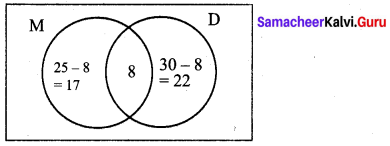(i) The number of students take part in only music is 17.
(ii) The number of students take part in only drama is 22.
(iii) The total number of students in the class is 17 + 8 + 22 = 47.

Samacheer Kalvi Guru 9th Maths Question 5.
In a party of 45 people, each one likes tea or coffee or both. 35 people like tea and 20 people like coffee. Find the number of people who
(i) like both tea and coffee.
(ii) do not like tea.
(iii) do not like coffee.
Solution:
Let the people who like tea be T.
Let the people who like coffee be C
By using formula
n( A ∪ B) = n(A) + n(B) – n(A ∩ B)

(i) n(T ∩ C) = n(T) + n(C) – n(T ∪ C) = 35 + 20 – 45 = 55 – 45 = 10
The number of people who like both coffee and tea = 10.

(ii) The number of people who do not like Tea
n(T) = n(U) – n(T) = 45 – 35 = 10

(iii) The number of people who do not like coffee
n(C’) = n(U) – n(C) = 45 – 20 = 25.

Samacheer Kalvi 9th Standard Maths Question 6.
In an examination 50% of the students passed in Mathematics and 70% of students passed in Science while 10% students failed in both subjects. 300 students passed in at least one subjects. Find the total number of students who appeared in the examination, if they took examination in only two subjects.
Solution:
Let the students who appeared in the examination be 100%.
Let the percentage of students who failed in mathematics be M.
Let the percentage of students who failed in science be S.
Failed in Maths = 100 % – Pass% = 100% – 50% = 50%
Failed in Science% 100% – 70% = 30%
Failed in both% = 10%
n(M ∪ S) = n(M) + n(S) – n(M ∩ S)
= 50% + 30% – 10% = 70%
% of students failed in atleast one subject = 70%
∴ The % of students who have passed in atleast one subject = 100% – 70% = 30%
30% = 300
∴ $$100 \%=\frac{100 \times 300}{30}=1000$$
∴ The total number of students who appeared in the examination = 1000 students.

Samacheer Kalvi 9th Maths Solutions Pdf Question 7.
A and B are two sets such that n(A – B) = 32 + x, n(B – A) = 5x and n(A ∩ B) = x. Illustrate the information by means of a venn diagram. Given that n(A) = n(B), calculate the value of x.
Solution: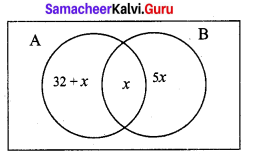n (A – B) = 32 +x
n(B – A) = 5x
n(A ∩ B) = x
n( A) = n(B)
32 + x + x = 5x + x
32 + 2x = 6x
4x = 32
x = 8

Samacheer Kalvi 9th Maths Question 8.
Out of 500 car owners investigated, 400 owned car A and 200 owned car B, 50 owned both A and B cars. Is this data correct?
Solution:
n( A ∪ B) = n(A) + n(B) – n( A ∩ B)
n(A ∪ B) = 500 (given) …………. (1).
n( A) = 400
n(B) = 200
n( A ∩ B) =50
∴ n(A ∩ B) = 400 + 200 – 50 = 550 …………… (2)
1 ≠ 2
∴ This data is incorrect.

9th Maths Samacheer Kalvi Question 9.
In a colony, 275 families buy Tamil newspaper, 150 families buy English newspaper, 45 families buy Hindi newspaper, 125 families buy Tamil and English newspapers, 17 families buy English and Hindi newspapers, 5 families buy Tamil and Hindi newspapers and 3 families buy all the three newspapers. If each family buy atleast one of these newspapers then find
(i) Number of families buy only one newspaper
(ii) Number of families buy atleast two newspapers
(iii) Total number of families in the colony.
Solution:
(i) Tamil Newspaper buyers n(A) = 275
English Newspaper buyers n(B) = 150
Hindi Newspaper buyers n(C) = 45
Tamil and English Newspaper buyers n(A ∩ B) = 125
English and Hindi Newspaper buyers n(B ∩ C) = 17
Hindi and Tamil Newspaper buyers n(C ∩ A) = 5
All the three Newspaper buyers n(A ∩ B ∩ C) = 3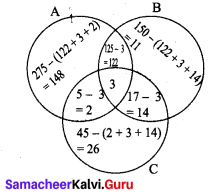(i) Number of families buy only one newspaper = 148 + 11 + 26 = 185
(ii) Number of families buy atleast two news papers = 122 + 14 + 2 + 3 = 141
(iii) Total number of families in the colony = 148 + 11 + 26 + 122 + 14 + 2 + 3 = 326

Question 10.
A survey of 1000 farmers found that 600 grew paddy, 350 grew ragi, 280 grew corn, 120 grew paddy and ragi, 100 grew ragi and corn, 80 grew paddy and corn. If each farmer grew atleast any one of the above three, then find the number of farmers who grew all the three.
Solution: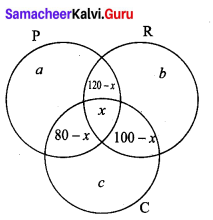a = 600 – (120 – x + x + 80 – x)
= 600 – (200 – x)
= 600 – 200 + x
= 400 + x
b = 350 – (120 – x + x + 100 – x)
= 350 – (220 – x)
= 350 – 230 + x
= 130 + x
c = 280 – (80 – x + x + 100 – x)
= 2800 – (180 – x) = 280 – 180 + x = 100 + x
Each farmer grew atleast one of the above three, the number of farmers who grew all the three is x.
= a + b + c + 120 – x + 100 – x + 80 – x + x = 1000
400 + x + 130 + x + 100 + x + 120 – x + 100 – x + 80 – x + x= 1000
∴ 930 + x = 1000
x = 1000 – 930 = 70
∴ 70 farmers grew all the three crops

9th Standard Maths Samacheer Kalvi Question 11.
In the adjacent diagram, if n(U) = 125,y is two times of x and z is 10 more than x, then find the value of x, y and z.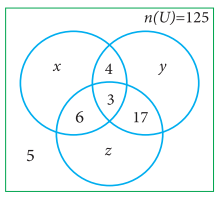Solution:
n(U) = 125
y = 2x
z = x + 10
∴ x + y + z + 4 + 17 + 6 + 3 + 5 = 125
x + 2x + x + 10 + 35 = 125
4x + 45 = 125
4x = 125 – 45
4x = 80
x = 20
∴ y = 2x = 2 × 20 = 40
z = x + 10 = 20 + 10 = 30
Hence x = 20 ; y = 40; z = 30

Samacheer Kalvi Guru Maths 9th Question 12.
Each student in a class of 35 plays atleast one game among chess, carrom and table tennis. 22 play chess, 21 play carrom, 15 play table tennis, 10 play chess and table tennis, 8 play carrom and table tennis and 6 play all the three games. Find the number of students who play (i) chess and carrom but not table tennis (ii) only chess (iii) only carrom (Hint: Use Venn diagram)
Solution: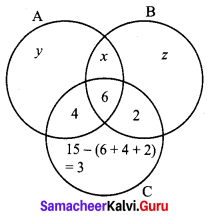A – Chess
B – Carrom
C – Table Tennis
n(A) = 22
n(B) = 21
n(C) = 15
n(A ∩ C) = 10
n(B ∩ C) = 8
n(A ∩ B ∩ C) = 6
(i) y = 22 – (x + 6 + 4) = 22 – (x + 10)
= 22 – x – 10
= 12 – x
z = 21 – (x + 6 + 2) = 21 – (8 + x)
21 – 8 – x = 13 – x
y + z + 3 + x + 2 + 4 + 6 = 35
12 – x + 13 – x + 15 + x = 35
40 – x = 35
x = 40 – 35 = 5
(i) Number of students who pay only chess and Carrom but not table tennis = 5
(ii) Number of students who play only chess = 12 – x = 12 – 5 = 7
(iii) Number of students who play only carrom = 13 – x = 13 – 5 = 8

9th Class Maths Exercise 1.6 Solution Question 13.
In a class of 50 students, each one come to school by bus or by bicycle or on foot. 25 by bus, 20 by bicycle, 30 on foot and 10 students by all the three. Now how many students come to school exactly by two modes of transport?
Solution: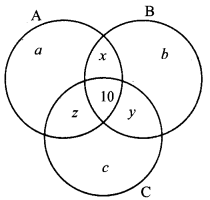A – by bus
B – by bicycle
C – on foot
n(A) = 25
n(B) = 20
n(C) = 30
n(A ∩ B ∩ C ) = 10
n(A ∪ B ∪ C ) = n(A) + n(B) + n(C) – n(A ∩ B) – n(B ∩ C) – n(C ∩ A) + n(A ∩ B ∩ C)
50 = 25 + 20 + 30 – (10 + x) – (10 + y) – (10 + z) + 10
50 = 75 – 10 – x – 10 – y – 10 – z + 10
= 75 – 20 – (x + y + z)
= 55 – (x + y + z)
x + y + z = 55 – 50 = 5
∴ The number of students who come to school exactly by two modes of transport = 5